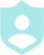###### Example QuestionsSolve each equation with the quadratic formula.Solve each equation with the quadratic formula.
##### Description :##### Difficulty :TRIVIA 10 QuestionsDownloadPrintShareAdd
Questions

•Solve each equation with the quadratic formula.Colors :
Font color
Button color
Background color
Logo:Background Image:Theme Song: